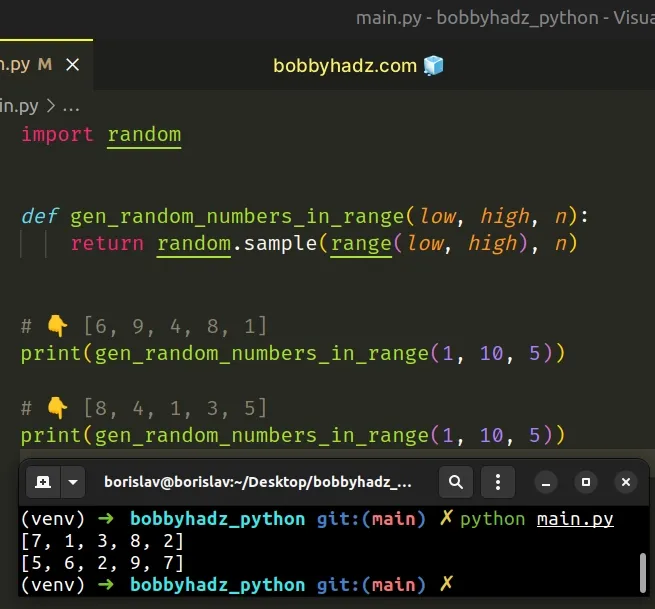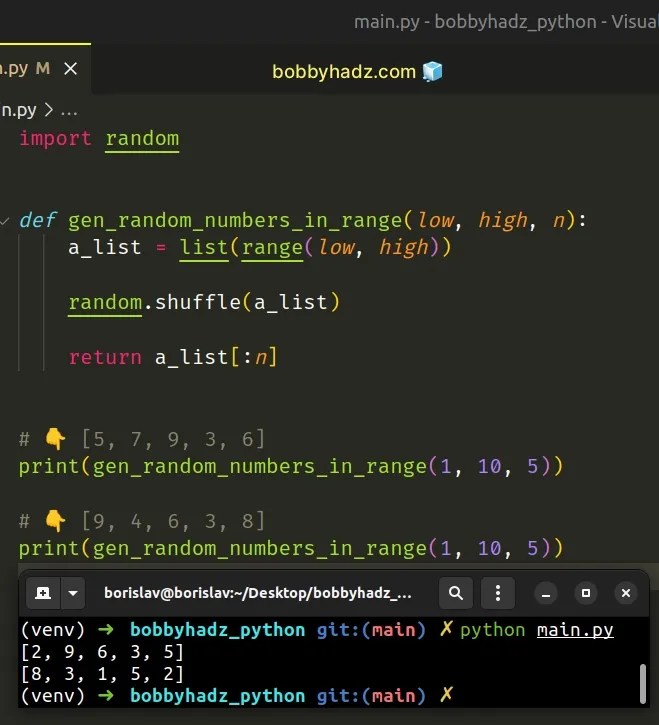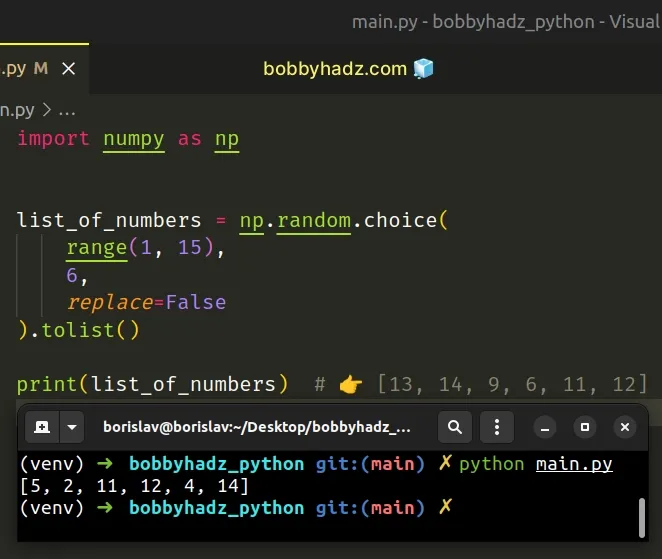# Generate N unique Random numbers within a Range in PythonLast updated: Feb 22, 2023
6 min## #Generate N unique random numbers within a range in Python

To generate N unique random numbers within a range:

1. Use the `range()` class to create a `range` object.
2. Use the `random.sample()` method to get a list of N unique random numbers.
3. The `random.sample()` method returns a list of N unique elements from the provided sequence.
main.py
```Copied!```import random

def gen_random_numbers_in_range(low, high, n):
return random.sample(range(low, high), n)

# 👇️ [6, 9, 4, 8, 1]
print(gen_random_numbers_in_range(1, 10, 5))

# 👇️ [8, 4, 1, 3, 5]
print(gen_random_numbers_in_range(1, 10, 5))
``````We used the `range()` class to get a `range` object.

main.py
```Copied!```print(list(range(1, 5)))  # 👉️ [1, 2, 3, 4]
print(list(range(1, 8)))  # 👉️ [1, 2, 3, 4, 5, 6, 7]
``````

The range class is commonly used for looping a specific number of times.

The `range()` class takes the following arguments:

NameDescription
`start`An integer representing the start of the range (defaults to `0`)
`stop`Go up to, but not including the provided integer
`step`Range will consist of every N numbers from `start` to `stop` (defaults to `1`)

The random.sample method returns a list of N unique elements chosen from the provided sequence.

The first argument the method takes is a sequence and the second is the number of random elements to be returned.
main.py
```Copied!```import random

# 👇️ [5, 3, 8, 4, 7]
print(
random.sample(
range(1, 10),
5
)
)
``````

The `random.sample()` method returns an N-sized list of elements chosen from the sequence without replacement.

Without replacement means that the same element cannot be returned multiple times.

The `random.sample()` method raises a `ValueError` if the sample is larger than the sequence.

main.py
```Copied!```import random

# ⛔️ ValueError
print(
random.sample(
range(1, 5),
5
)
)
``````

If you need to handle this scenario, use a try/except block.

main.py
```Copied!```import random

try:
random_numbers = random.sample(
range(1, 5),
5
)
except ValueError:
# 👇️ this runs
print('The sample is larger than the sequence')
``````

Trying to retrieve more elements than are present in the `range` caused a `ValueError` which was then handled by the `except` block.

Alternatively, you can use the `random.shuffle()` method.

## #Generate N unique random numbers within a range using random.shuffle()

This is a four-step process:

1. Use the `range()` class to get a `range` object.
2. Use the `list()` class to convert the `range` object to a list.
3. Use the `random.shuffle()` method to shuffle the list.
4. Use list slicing to get N unique random numbers from the list.
main.py
```Copied!```import random

def gen_random_numbers_in_range(low, high, n):
a_list = list(range(low, high))

random.shuffle(a_list)

return a_list[:n]

# 👇️ [5, 7, 9, 3, 6]
print(gen_random_numbers_in_range(1, 10, 5))

# 👇️ [9, 4, 6, 3, 8]
print(gen_random_numbers_in_range(1, 10, 5))
``````The random.shuffle method takes a sequence and shuffles it in place.

main.py
```Copied!```import random

a_list = list(range(1, 10))

random.shuffle(a_list)

print(a_list)  # 👉️ [4, 7, 3, 9, 1, 2, 8, 6, 5]
``````

The last step is to use list slicing to select N unique random elements from the list.

The syntax for list slicing is `my_list[start:stop:step]`.

The `start` index is inclusive and the `stop` index is exclusive (up to, but not including).

If the `start` index is omitted, it is considered to be `0`, if the `stop` index is omitted, the slice goes to the end of the list.

Python indexes are zero-based, so the first item in a list has an index of `0`, and the last item has an index of `-1` or `len(my_list) - 1`.

The slice `list[:n]` returns the first `n` elements of the shuffled list.

## #Create list of random numbers without duplicates using NumPy

This is a three-step process:

1. Use the `range()` class to create a `range` object.
2. Use the `numpy.random.choice()` method to create a list of numbers without duplicates.
3. Set the `replace` argument to `False` to select unique numbers.
main.py
```Copied!```import numpy as np

list_of_numbers = np.random.choice(
range(1, 15),
6,
replace=False
).tolist()

print(list_of_numbers)  # 👉️ [13, 14, 9, 6, 11, 12]
``````Make sure you have the NumPy module installed to be able to run the code sample.

shell
```Copied!```pip install numpy

# 👇️ or with pip3
pip3 install numpy
``````

The numpy.random.choice method generates a random sample from the given array-like object.

The `replace` argument determines whether a value of the array-like object can be selected multiple times. The argument defaults to `True`.

We set the `replace` argument to `False`, so no duplicates are selected.

You can use the tolist method if you need to convert the NumPy array to a native Python list.

## #Generate random number in range excluding some numbers

To generate a random number in a range, excluding some numbers:

1. Use a list comprehension to iterate over a `range` object.
2. Use the `not in` operator to exclude the numbers from the list.
3. Use the `random.choice()` method to generate a random number in the range.
main.py
```Copied!```from random import choice

def gen_random_number(low, high, exclude):
return choice(
[number for number in range(low, high)
if number not in exclude]
)

numbers_to_exclude = [1, 3, 7]

# 👇️ 2
print(gen_random_number(1, 10, numbers_to_exclude))

# 👇️ 19
print(gen_random_number(1, 100, numbers_to_exclude))
``````

We used a list comprehension to iterate over a `range` object.

List comprehensions are used to perform some operation for every element or select a subset of elements that meet a condition.

The range class is commonly used for looping a specific number of times.

main.py
```Copied!```print(list(range(1, 5))) # 👉️ [1, 2, 3, 4]
print(list(range(1, 3))) # 👉️ [1, 2]
``````

On each iteration, we use the `not in` operator to exclude a list of numbers from the result.

main.py
```Copied!```from random import choice

def gen_random_number(low, high, exclude):
return choice(
[number for number in range(low, high)
if number not in exclude]
)
``````

The in operator tests for membership. For example, `x in l` evaluates to `True` if `x` is a member of `l`, otherwise, it evaluates to `False`.

`x not in l` returns the negation of `x in l`.

The last step is to use the `random.choice()` method.

The random.choice method takes a sequence and returns a random element from the non-empty sequence.

main.py
```Copied!```import random

print(random.choice(['a', 'b'])) # 👉️ "a"
``````

If the sequence is empty, the method raises an `IndexError`.

If you need to generate N random numbers in a range, excluding a list of numbers, use a list comprehension.
main.py
```Copied!```from random import choice

def gen_random_number(low, high, exclude):
return choice(
[number for number in range(low, high)
if number not in exclude]
)

numbers_to_exclude = [1, 3, 7]

result = [
gen_random_number(1, 10, numbers_to_exclude) for _ in range(3)
]
print(result)  # 👉️ [5, 2, 5]
``````

We used a list comprehension to iterate over a `range` object of length N and called the `get_random_number()` function on each iteration.

Alternatively, you can use `set` objects.

## #Generate random number in range excluding some numbers using a set

This is a four-step process:

1. Get a `set` containing all numbers in the range.
2. Convert the list of numbers to exclude to a `set` object.
3. Get the difference between the `set` objects.
4. Use the `random.choice()` method to generate a random number in the range.
main.py
```Copied!```from random import choice

def gen_random_number(low, high, exclude):
return choice(
list(
set(number for number in range(low, high)) -
set(exclude)
)
)

numbers_to_exclude = [1, 3, 7]

print(gen_random_number(1, 10, numbers_to_exclude))  # 👉️ 4

result = [
gen_random_number(1, 10, numbers_to_exclude) for _ in range(3)
]
print(result)  # 👉️ [5, 2, 5]
``````

We used the set() class to convert the `range` object and the list of numbers to exclude to `set` objects.

Set objects are an unordered collection of unique elements and implement a `difference()` method.

The minus sign is a shorthand for calling the `difference()` method on the `set`.

main.py
```Copied!```print({1, 2, 3} - {2, 3}) # 👉️ {1}
``````

The difference() method returns a new `set` with elements in the `set` that are not in the provided iterable.

We used the list() class to convert the `set` object to a list and used the `random.choice()` method to pick a random number from the list.